## 9.4.2 Greek Letters

Greek letters that differ from the corresponding Roman letters are obtained by placing a backslash in front of the name.9.1 Lower case and upper case Greek letters are shown in Table 9.3 and Table 9.4, respectively. There are also some variants of certain symbols, such as \vartheta as opposed to \theta.

 \alpha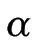\beta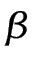\gamma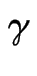\delta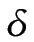\epsilon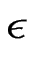\varepsilon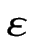\zeta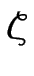\eta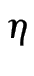\theta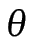\vartheta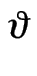\iota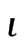\kappa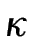\lambda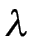\mu\nu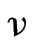\xi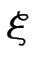\pi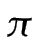\varpi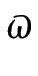\rho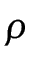\varrho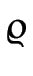\sigma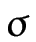\varsigma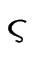\tau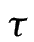\upsilon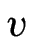\phi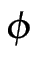\varphi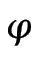\chi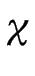\psi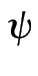\omega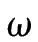\Gamma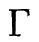\Delta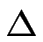\Theta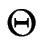\Lambda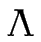\Xi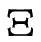\Pi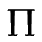\Sigma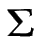\Upsilon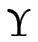\Phi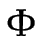\Psi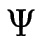\Omega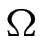Example:

The following code

$x' = x + \Delta x$

produces:#### Footnotes

... name.9.1
So, for example, there is no omicron since it looks the same as a Roman o.

This book is also available as A4 PDF or 12.8cm x 9.6cm PDF or paperback (ISBN 978-1-909440-00-5).

© 2012 Dickimaw Books. "Dickimaw", "Dickimaw Books" and the Dickimaw parrot logo are trademarks. The Dickimaw parrot was painted by Magdalene Pritchett.